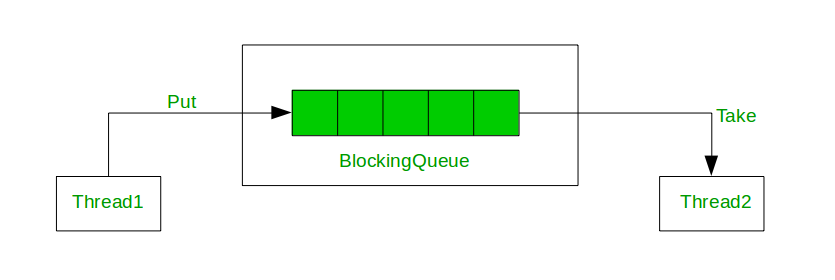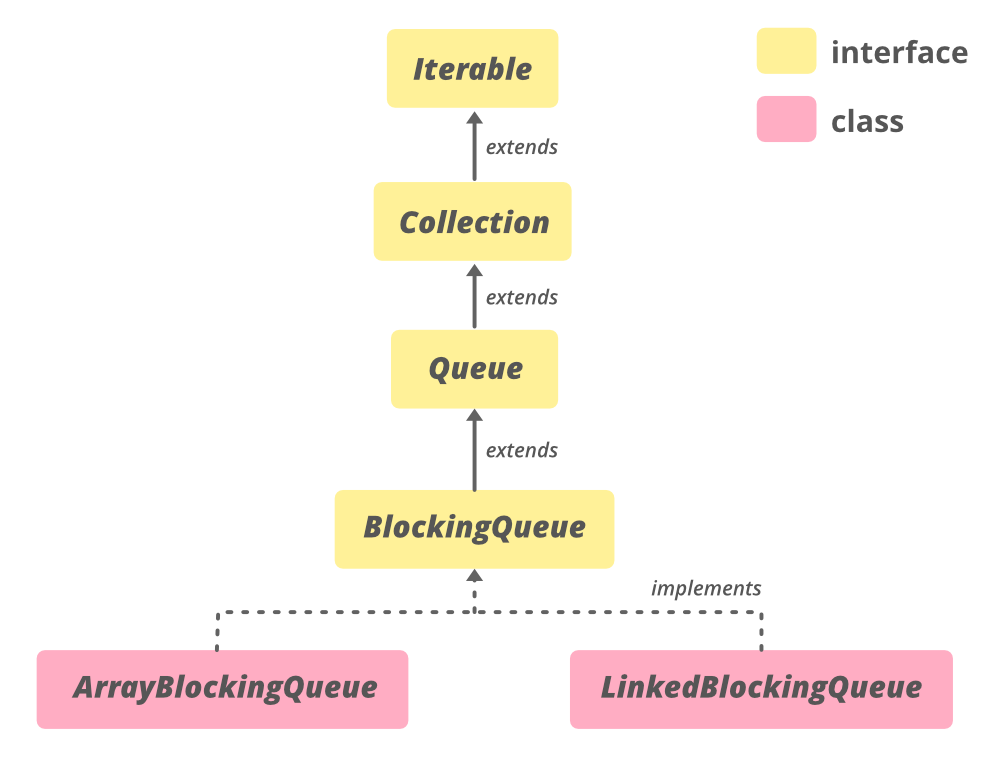Related Articles
BlockingQueue Interface in Java
• Difficulty Level : Easy
• Last Updated : 21 Oct, 2020

The BlockingQueue interface in Java is added in Java 1.5 along with various other concurrent Utility classes like ConcurrentHashMap, Counting Semaphore, CopyOnWriteArrrayList, etc. BlockingQueue interface supports flow control (in addition to queue) by introducing blocking if either BlockingQueue is full or empty. A thread trying to enqueue an element in a full queue is blocked until some other thread makes space in the queue, either by dequeuing one or more elements or clearing the queue completely. Similarly, it blocks a thread trying to delete from an empty queue until some other treads insert an item. BlockingQueue does not accept a null value. If we try to enqueue the null item, then it throws NullPointerException.

Java provides several BlockingQueue implementations such as LinkedBlockingQueue, ArrayBlockingQueue, PriorityBlockingQueue, SynchronousQueue, etc. Java BlockingQueue interface implementations are thread-safe. All methods of BlockingQueue are atomic in nature and use internal locks or other forms of concurrency control. Java 5 comes with BlockingQueue implementations in the java.util.concurrent package

Usage of BlockingQueueThe Hierarchy of BlockingQueueDeclaration

```public interface BlockingQueue<E> extends Queue<E>
```

Here, E is the type of elements stored in the Collection.

### Classes that Implement BlockingQueue

We directly cannot provide an instance of BlockingQueue since it is an interface, so to utilize the functionality of the BlockingQueue, we need to make use of the classes implementing it. Also, to use BlockingQueue in your code, use this import statement.

```import java.util.concurrent.BlockingQueue;
(or)
import java.util.concurrent.*;
```

The implementing class of BlockingDeque is LinkedBlockingDeque. This class is the implementation of the BlockingDeque and the linked list data structure. The LinkedBlockingDeque can be optionally bounded using a constructor, however, if the capacity is unspecified it is Integer.MAX_VALUE by default. The nodes are added dynamically at the time of insertion obeying the capacity constraints.

The syntax for creating objects:

```BlockingQueue<?> objectName = new LinkedBlockingDeque<?>();
(or)
```

Example: In the code given below we perform some basic operations on a LinkedBlockingDeque, like creating an object, adding elements, deleting elements, and using an iterator to traverse through the LinkedBlockingDeque.

### BlockingQueue Types

The BlockingQueue are two types:

1. Unbounded Queue: The Capacity of the blocking queue will be set to Integer.MAX_VALUE. In the case of an unbounded blocking queue, the queue will never block because it could grow to a very large size. when you add elements its size grows.

Syntax:

```BlockingQueue blockingQueue = new LinkedBlockingDeque();
```

2. Bounded Queue: The second type of queue is the bounded queue. In the case of a bounded queue you can create a queue passing the capacity of the queue in queues constructor:

Syntax:

```// Creates a Blocking Queue with capacity 5
```

To implement Bounded Semaphore using BlockingQueue

## Java

 `// Java program that explains the internal``// implementation of BlockingQueue`` ` `import` `java.io.*;``import` `java.util.*;`` ` `class` `BlockingQueue {`` ` `    ``// BlockingQueue using LinkedList structure``    ``// with a constraint on capacity``    ``private` `List queue = ``new` `LinkedList();`` ` `    ``// limit variable to define capacity``    ``private` `int` `limit = ``10``;`` ` `    ``// constructor of BlockingQueue``    ``public` `BlockingQueue(``int` `limit) { ``this``.limit = limit; }`` ` `    ``// enqueue method that throws Exception``    ``// when you try to insert after the limit``    ``public` `synchronized` `void` `enqueue(E item)``        ``throws` `InterruptedException``    ``{``        ``while` `(``this``.queue.size() == ``this``.limit) {``            ``wait();``        ``}``        ``if` `(``this``.queue.size() == ``0``) {``            ``notifyAll();``        ``}``        ``this``.queue.add(item);``    ``}`` ` `    ``// dequeue methods that throws Exception``    ``// when you try to remove element from an``    ``// empty queue``    ``public` `synchronized` `E dequeue()``        ``throws` `InterruptedException``    ``{``        ``while` `(``this``.queue.size() == ``0``) {``            ``wait();``        ``}``        ``if` `(``this``.queue.size() == ``this``.limit) {``            ``notifyAll();``        ``}`` ` `        ``return` `this``.queue.remove(``0``);``    ``}``   ` `    ``public` `static` `void` `main(String []args)``    ``{``    ``}``}`

Example:

## Java

 `// Java Program to demonstrate usuage of BlockingQueue`` ` `import` `java.util.concurrent.*;``import` `java.util.*;`` ` `public` `class` `GFG {`` ` `    ``public` `static` `void` `main(String[] args)``        ``throws` `InterruptedException``    ``{`` ` `        ``// define capacity of ArrayBlockingQueue``        ``int` `capacity = ``5``;`` ` `        ``// create object of ArrayBlockingQueue``        ``BlockingQueue queue``            ``= ``new` `ArrayBlockingQueue(capacity);`` ` `        ``// Add elements to ArrayBlockingQueue using put``        ``// method``        ``queue.put(``"StarWars"``);``        ``queue.put(``"SuperMan"``);``        ``queue.put(``"Flash"``);``        ``queue.put(``"BatMan"``);``        ``queue.put(``"Avengers"``);`` ` `        ``// print Queue``        ``System.out.println(``"queue contains "` `+ queue);`` ` `        ``// remove some elements``        ``queue.remove();``        ``queue.remove();`` ` `        ``// Add elements to ArrayBlockingQueue``        ``// using put method``        ``queue.put(``"CaptainAmerica"``);``        ``queue.put(``"Thor"``);`` ` `        ``System.out.println(``"queue contains "` `+ queue);``    ``}``}`
Output:
```queue contains [StarWars, SuperMan, Flash, BatMan, Avengers]
queue contains [Flash, BatMan, Avengers, CaptainAmerica, Thor]```

### Basic Operations

Elements can be added into a LinkedBlockedDeque in different ways depending on the type of structure we are trying to use it as. The most common method is the add() method using which we can add elements at the end of the deque. We can also use the addAll() method (which is a method of the Collection interface) to add an entire collection to LinkedBlockingDeque. If we wish to use the deque as a queue we can use add() and put().

## Java

 `// Java Program Demonstrate add()``// method of BlockingQueue`` ` `import` `java.util.concurrent.LinkedBlockingDeque;``import` `java.util.concurrent.BlockingQueue;``import` `java.util.*;`` ` `public` `class` `GFG {``   ` `    ``public` `static` `void` `main(String[] args)``        ``throws` `IllegalStateException``    ``{`` ` `        ``// create object of BlockingQueue``        ``BlockingQueue BQ``            ``= ``new` `LinkedBlockingDeque();`` ` `        ``// Add numbers to the BlockingQueue``        ``BQ.add(``7855642``);``        ``BQ.add(``35658786``);``        ``BQ.add(``5278367``);``        ``BQ.add(``74381793``);`` ` `        ``// before removing print BlockingQueue``        ``System.out.println(``"Blocking Queue: "` `+ BQ);``    ``}``}`
Output
`Blocking Queue: [7855642, 35658786, 5278367, 74381793]`

2. Accessing Elements

The elements of the LinkedBlockingDeque can be accessed using contains(), element(), peek(), poll(). There are variations of these methods too which are given in the table above along with their descriptions.

## Java

 `// Java Program for Accessing the elements of a``// LinkedBlockingDeque`` ` `import` `java.util.concurrent.*;`` ` `public` `class` `AccessingElements {`` ` `    ``public` `static` `void` `main(String[] args)``    ``{`` ` `        ``// Instantiate an object of LinkedBlockingDeque``        ``// named lbdq``        ``BlockingQueue lbdq``            ``= ``new` `LinkedBlockingDeque();`` ` `        ``// Add elements using add()``        ``lbdq.add(``22``);``        ``lbdq.add(``125``);``        ``lbdq.add(``723``);``        ``lbdq.add(``172``);``        ``lbdq.add(``100``);`` ` `        ``// Print the elements of lbdq on the console``        ``System.out.println(``            ``"The LinkedBlockingDeque, lbdq contains:"``);``        ``System.out.println(lbdq);`` ` `        ``// To check if the deque contains 22``        ``if` `(lbdq.contains(``22``))``            ``System.out.println(``                ``"The LinkedBlockingDeque, lbdq contains 22"``);``        ``else``            ``System.out.println(``"No such element exists"``);`` ` `        ``// Using element() to retrieve the head of the deque``        ``int` `head = lbdq.element();``        ``System.out.println(``"The head of lbdq: "` `+ head);``    ``}``}`
Output
```The LinkedBlockingDeque, lbdq contains:
[22, 125, 723, 172, 100]

3. Deleting Elements

Elements can be deleted from a LinkedBlockingDeque using remove(). Other methods such as take() and poll() can also be used in a way to remove the first and the last elements.

## Java

 `// Java Program for removing elements from a``// LinkedBlockingDeque`` ` `import` `java.util.concurrent.*;`` ` `public` `class` `RemovingElements {`` ` `    ``public` `static` `void` `main(String[] args)``    ``{`` ` `        ``// Instantiate an object of LinkedBlockingDeque``        ``// named lbdq``        ``BlockingQueue lbdq``            ``= ``new` `LinkedBlockingDeque();`` ` `        ``// Add elements using add()``        ``lbdq.add(``75``);``        ``lbdq.add(``86``);``        ``lbdq.add(``13``);``        ``lbdq.add(``44``);``        ``lbdq.add(``10``);`` ` `        ``// Print the elements of lbdq on the console``        ``System.out.println(``            ``"The LinkedBlockingDeque, lbdq contains:"``);``        ``System.out.println(lbdq);`` ` `        ``// Remove elements using remove();``        ``lbdq.remove(``86``);``        ``lbdq.remove(``44``);`` ` `        ``// Trying to remove an element``        ``// that doesn't exist``        ``// in the LinkedBlockingDeque``        ``lbdq.remove(``1``);`` ` `        ``// Print the elements of lbdq on the console``        ``System.out.println(``            ``"\nThe LinkedBlockingDeque, lbdq contains:"``);``        ``System.out.println(lbdq);``    ``}``}`
Output
```The LinkedBlockingDeque, lbdq contains:
[75, 86, 13, 44, 10]

[75, 13, 10]```

4. Iterating through the Elements

To iterate through the elements of a LinkedBlockingDeque we can create an iterator and use the methods of the Iterable interface, which is the root of the Collection Framework of Java, to access the elements. The next() method of Iterable returns the element of any collection.

## Java

 `// Java Program to iterate ``// through the LinkedBlockingDeque``import` `java.util.Iterator;``import` `java.util.concurrent.*;`` ` `public` `class` `IteratingThroughElements {`` ` `    ``public` `static` `void` `main(String[] args) {``         ` `        ``// Instantiate an object of LinkedBlockingDeque named lbdq``        ``BlockingQueue lbdq = ``new` `LinkedBlockingDeque();``         ` `        ``// Add elements using add()``        ``lbdq.add(``166``);``        ``lbdq.add(``246``);``        ``lbdq.add(``66``);``        ``lbdq.add(``292``);``        ``lbdq.add(``98``);``         ` `        ``// Create an iterator to traverse lbdq``        ``Iterator lbdqIter = lbdq.iterator();``         ` `        ``// Print the elements of lbdq on to the console``        ``System.out.println(``"The LinkedBlockingDeque, lbdq contains:"``);``         ` `        ``for``(``int` `i = ``0``; i
Output
```The LinkedBlockingDeque, lbdq contains:
166 246 66 292 98 ```

### The Behavior of BlockingQueue Methods

The following are the methods provided by the BlockingQueue for insertion, removal, and examine operations on the queue. Each of the four sets of methods behaves differently if the requested operation is not satisfied immediately.

• Throws Exception: An exception will be thrown, if the requested operation is not satisfied immediately.
• Special value: A special value is returned if the operation is not satisfied immediately.
• Blocks: The method call is blocked if the attempted operation is not satisfied immediately and it waits until it gets executed.
• Times out: A special value is returned telling whether the operation succeeded or not. If the requested operation is not possible immediately, the method call blocks until it is, but waits no longer than the given timeout.

Attention reader! Don’t stop learning now. Get hold of all the important Java Foundation and Collections concepts with the Fundamentals of Java and Java Collections Course at a student-friendly price and become industry ready. To complete your preparation from learning a language to DS Algo and many more,  please refer Complete Interview Preparation Course.

My Personal Notes arrow_drop_up